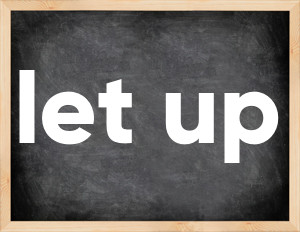# Phrasal verb let upEnglish verb let up [let ʌp].
Relate to: phrasal verb.
3 forms of verb let up: Infinitive (let up), Past Simple - (let up), Past Participle - (let up).

## Forms of verb let up in past tenses

👉 Forms of verb let up in future and past simple and past participle.
❓ What is the past tense of let up.

## Three forms of verb let up

Base Form Past Simple Past Participle
let up [let ʌp]

let up [let ʌp]

let up [let ʌp]

## What is 2nd and 3rd form of let up?

🎓 Base Form (Infinitive) let up in Past Simple, Future Simple, Present Perfect, Past Perfect, Future Perfect?

• First form (V1) - let up. (Present simple, Future Simple)
• Second form (V2) - let up. (Past simple)
• Third form (V3) - let up. (Present perfect, Past perfect)

## What are the past tense and past participle of let up?

The past tense of let up is: let up in past simple is let up. and past participle is let up.

### What is the past tense of let up?

The past tense of let up is let up.
The past participle of let up is let up.

### Verb Tenses

Past simple — let up in past simple let up. (V2)
Future simple — let up in future simple let up. (will + V1)
Present Perfect — let up in present perfect tense let up. (have\has + V3)
Past Perfect — let up in past perfect tense let up. (had + V3)

### let up regular or irregular verb?

👉 Is let up an irregular verb?? let up is .

## Examples of Verb let up in Sentences

•   Stop wrestling your brother and let him up (Present Simple)
•   Don't let up the suspect until you've put handcuffs on him (Present Simple)
•   I told the receptionist not to let up anyone he didn't recognize (Past Simple)
•   I'm hoping the rain lets up soon (Present Simple)
•   The snow hasn't let up for nearly a week (Present Perfect)
•   Let up on the accelerator a little, or you'll burn out the engine (Present Simple)
•   They are not going to let up on us in the second half (Present Simple)
•   When the snow lets up so I can see, I will drive to the store (Future Simple)

Along with let up, words are popular settle for and live with.

Verbs by letter: , , , , , , , , , , , , , , , , , , , , , , , , .